# Solve a Very Old ProblemOver 2000 years ago the Greek mathematician Euclid came up with a complete set of rules (called axioms) that described everything you could possible do with a straight edge (a ruler with no marks) and a compass on a flat piece of paper. (You can read more about these in Axioms of Geometry.)

On of the useful things you can do with a straight edge and compass, is to divide any angle in two.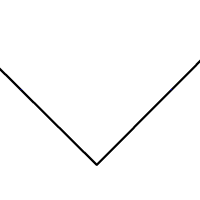If you can divide an angle in two, called bisecting an angle, you might think the next step would be to trisect an angle, that is, to divide it into three. But try as he might, Euclid and every other mathematician in the centuries since could not manage it.

The problem of whether angle trisection could be done in the general case remained a mathematical mystery for millennia. Many many mathematicians, both amateur and professional, have tried. It was finally shown, in 1837, that it was impossible by Pierre Wantzel, a French mathematician and expert on arithmetic. (Wantzel was a promising mathematician, proving this result when he was just 23. But sadly he died tragically young, at the age of 33.)

So why is it impossible? Pierre showed that the two problems of trisecting an angle and of solving a cubic equation are equivalent (you can read why this is true here). Moreover, he showed that only a very few cubic equations can be solved using the straight-edge-and-compass method – most cannot. And so he realised that most angles cannot be trisected.

This hasn’t stopped people trying – at Plus we still occasionally get letters from people saying they’ve finally found the way to trisect an angle using Euclid’s tools. But that’s not really surprising as it’s a very tricky concept that you can prove something is impossible, and it might seem very strange indeed that the reason it is impossible links angles and geometry to equations.

But we can trisect an angle if we use a different set of tools. Euclid’s axioms aren’t the only ones that describe what you can do on a flat piece of paper: we also have the axioms of origami. And it turns out that folding paper with one single flat fold at a time is more powerful than using a straight edge and compass.

So, how do you trisect an angle with origami?

First of all mark out your angle. You can do this with a single fold running through the bottom corner of the paper.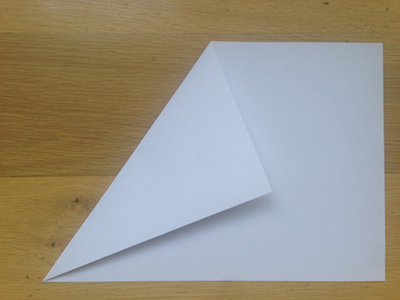Here is the angle we'd like to trisect: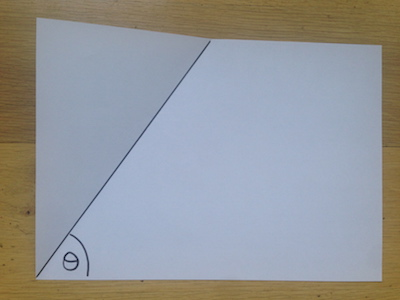The first step is to make a fold that is parallel to the bottom edge of the paper, as the bottom edge represents one of the boundaries of our angle. You want this fold to be somewhere in the top half of the piece of paper. So pick a point in the top half and make a fold through that point so that the fold is perpendicular to the side of the sheet (by neatly folding the side of the sheet onto itself). This way, your fold is parallel to the top and bottom of the paper.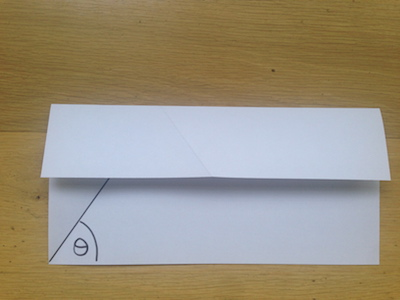Now fold up the bottom of the paper to meet your horizontal fold in the top half of the sheet.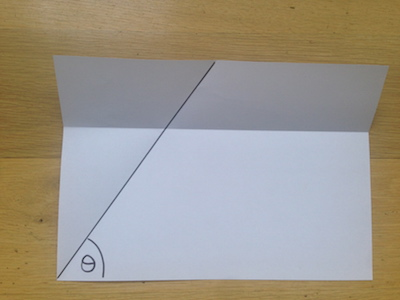You should now have two horizontal folds, parallel to the bottom of the paper.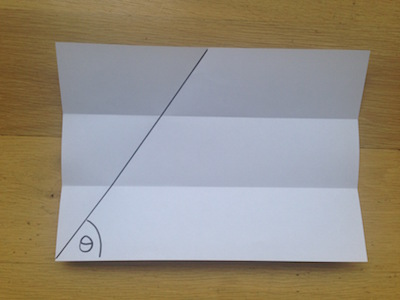The next step is a little trickier, but we know it’s possible thanks to the axioms of origami. You want to make a fold that matches two points to two lines. You want the point where your upper horizontal fold meets the edge of the paper to end up on the angled line marking the boundary of your angle. And you want the bottom left corner of the paper to end up on your lower horizontal fold. It takes a bit of trial and error to get the placing of the fold right before you make the final crease, but persevere because you can do it!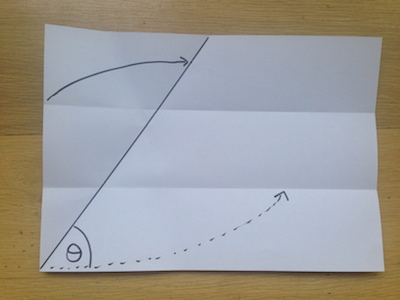Phew! Here is what it should look like: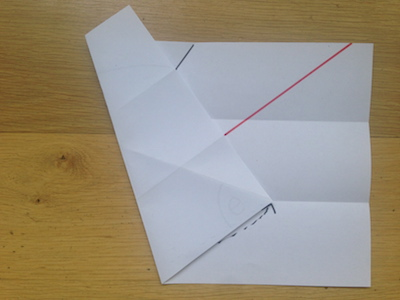You’ll notice that now the lower horizontal fold runs at an angle up the page on the part that has been folded over, indicated in red in the picture. Fold along this angled line.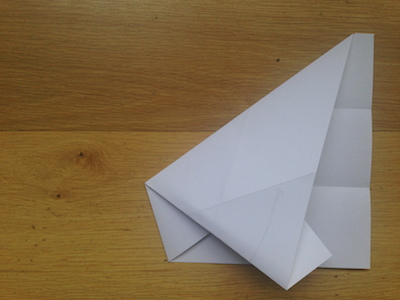Now if you open the sheet out you’ll see that this has produced a fold that runs toward the bottom left corner, within the boundaries of our original angle.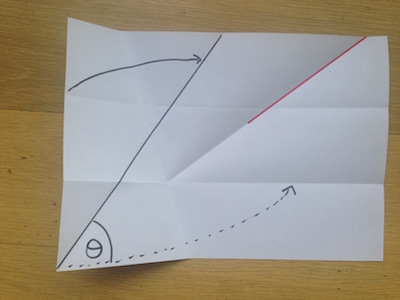Now that the paper is opened out, make that fold again so it runs all the way to the corner.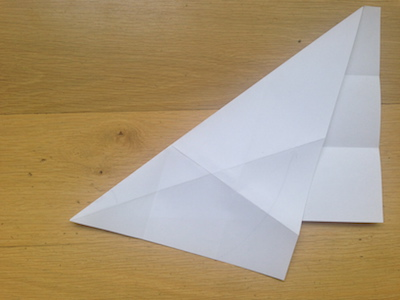You should now have this: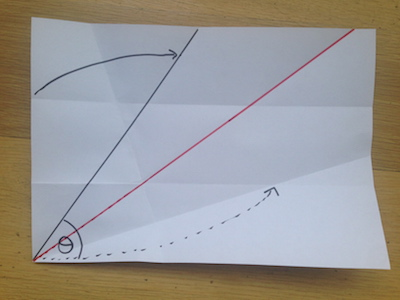Nearly there! The final step is to fold the bottom edge of the paper up to meet this new fold.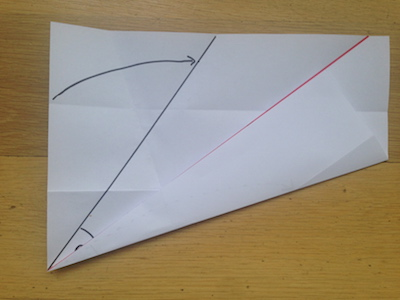Open it up and hey presto... you’ve trisected the angle! You’ve solved a millennia old problem with a few folds!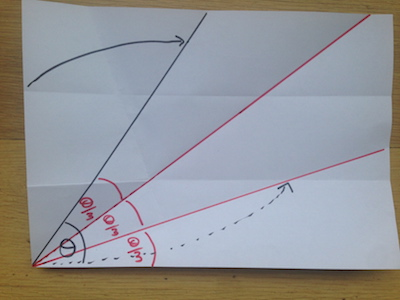You can read more about the power of origami and a proof of why this works.